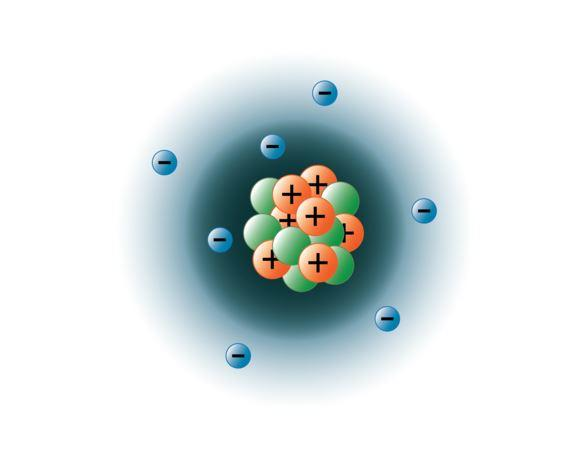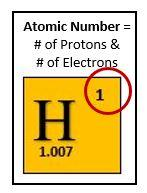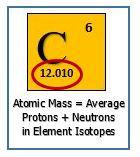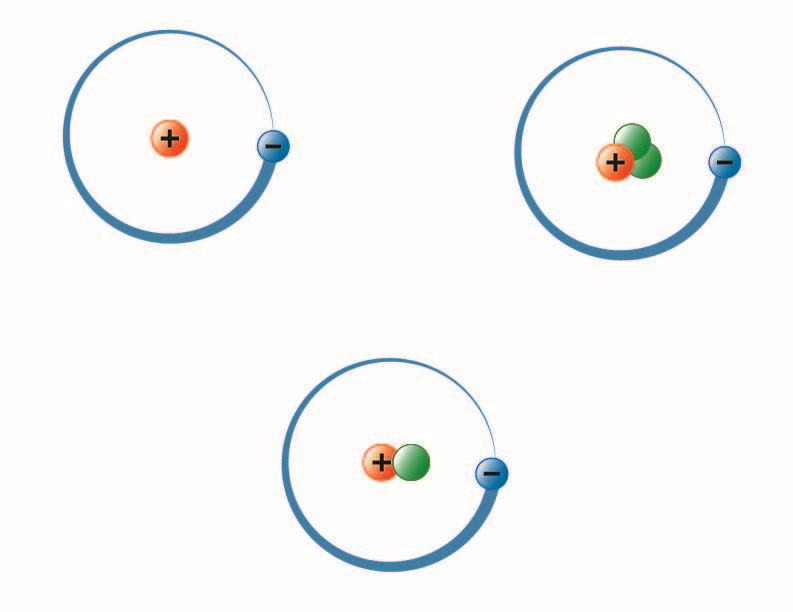1.1.1

# Subatomic Particles

All elements are composed of extremely small particles of matter called atoms. We can define an atom as the simplest particle of an element that still has the chemical properties of that element. Chemical properties include the physical state of the element during standard conditions (gas, liquid, or solid), the types of bonds the element can form, how it reacts with other elements, etc. For example, all carbon atoms have the same chemical properties.

Physicists have succeeded in blasting atoms apart into dozens of different sub-atomic particles; however, only three of them are stable. These stable sub-atomic particles are the protons, neutrons, and electrons. Protons are particles that are positively charged, have mass, and are in the center (or nucleus) of that atom. Neutrons also have mass and are located in the nucleus but have not charge. Neutrons bind with protons in a way that helps stabilize the nucleus. Too many or too few neutrons may result in an atomic nucleus that is unstable and may decay to form other elements. We refer to these types of unstable atoms as being radioactive. Although the mass of the neutron is slightly greater than that of a proton, we can assign both the relative mass of 1 (1 atomic mass unit or amu). We use the atomic mass unit because the actual weight of a proton is 1.67 x 10-24 grams. The AMU is measured in Daltons, named after John Dalton who mathematically showed that a unit Dalton is approximately equal to the molar mass of the same element in grams per mole (1AMU = 1 Dalton).

Neutrons and protons constitute almost all of an atom’s mass. The third type of stable particle is the electron. Electrons have a negative charge but are extremely small and have a mass only 1/1850 that of a proton or neutron. They are so small that, for practical purposes, they do not contribute to the mass of the atom, thus are assigned a 0 for their amu. Electrons move around the nucleus at tremendously high speeds traveling near the speed of light. Although we often describe electrons as residing in orbits that circle the nucleus, like planets orbiting the sun, modern physics teaches us that this model is incorrect. These “orbitals” are areas in space around the nucleus where the electrons will be located most of the time. This area is often referred to as the electron “cloud.” True, it is still a specific area, but it is a bit more amorphous (without a clearly defined shape or form) than a spherical orbit. For simplicity, however, we often think of these as satellite-like circular orbitals. The image below represents our current model of a nitrogen atom.

The nitrogen nucleus contains 7 protons (orange) and 7 neutrons (green). The shaded areas around the nucleus represent the electron orbitals (clouds). Electrons (blue) will be found somewhere within these orbitals. (Note: the image is not drawn to scale. It has been suggested that if the nucleus were the size of a basketball, the electrons would be about six kilometers or 3¾ miles away!)####Take a look at the periodic table again and notice the number at the top of each box. This number is the atomic number and is unique for each element. For example, the atomic number for hydrogen is 1, indicating it has 1 proton. No other element has an atomic number of 1.  For carbon, the atomic number is 6 and, again, no other element has an atomic number of 6. The significance of the atomic number is that it tells us the number of protons in the nucleus of each element. Therefore, all hydrogen atoms have 1 proton (which is why the term hydrogen ion is sometimes used as a substitute for the word proton), and all carbon atoms have 6 protons. Note: if you change the number of protons, you change the actual element. In addition, since atoms have a neutral charge, the atomic number also tells us the number of electrons in the atom. In chemical notation, the atomic number for an element is expressed as a subscript preceding the symbol for the element. For example, carbon would be expressed as 6C.

#### Mass Number (Atomic Mass)The mass number of an atom, as the name implies, tells the total mass of the atom. Since the mass of an electron is extremely small (negligible), it isn’t used in the computation of the mass number. Also, recall that the mass of each proton, as well as each neutron, is 1 atomic mass unit. Therefore, the mass number is the sum of the protons and neutrons in the atom.

Since the mass number is the number of protons plus the number of neutrons and the atomic number is the number of protons, you can find the number of neutrons by simply subtracting the atomic number from the mass number. As an example, suppose we have an element with an atomic number of 8 and a mass number of 17. From this information, you can deduce that this element has 8 protons, 8 electrons, and 9 neutrons (17-8=9).

Now, let’s throw you a curve ball. As mentioned above, all atoms of a given element have the same number of protons (atomic number). However, different atoms of a given element may have different numbers of neutrons. When this happens, we call these elements isotopes. For example, there are three isotopes of hydrogen. The most common isotope comprising 99.98% of all hydrogen atoms has a mass number of 1. It, therefore, is composed of one proton, no neutrons, and one electron. The other less abundant isotopes of hydrogen have mass numbers of 2 and 3, respectively. These isotopes differ in the number of neutrons in their nuclei, but all three have one proton and one electron. There are naturally occurring isotopes of every element, each having its own unique mass number. In chemical notation, the mass number for a given isotope is expressed as a superscript preceding the symbol for the element. The three isotopes for hydrogen would be expressed as 1H, 2H, and 3H.

Since each element is composed of several isotopes, one question that arises is “what is the actual mass of a given element?” Again, if you look at the periodic table above, you will notice a number in the bottom of each box. This is the atomic weight for the element. For example, the atomic weight of hydrogen is 1.00794 amu. This number was derived by computing the average mass of the 3 isotopes of hydrogen. Since 1H is the most abundant isotope of hydrogen, it makes sense that the average atomic weight for hydrogen is very close to the atomic mass of 1H.The image above represents the three isotopes of hydrogen. The most common (upper left) has one proton and no neutrons in the nucleus. Deuterium (bottom) has one proton and one neutron, and Tritium (upper right) has one proton and two neutrons.